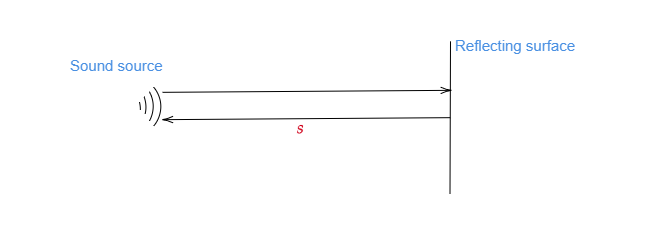# The echo of a sound is heard after 5 seconds. If the speed of sound in air be 342 m/s, calculate the distance of the reflecting surface.

Let $s$ be the distance between the sound source and the reflecting surface. For echo, it is necessary that it travels $2s$ distance as illustrated in the figure:Here time is taken $t=5\ seconds$

Speed of the sound $=342\ m/s$

We know the speed distance formula:

$distance=speed\times time$

Or $2s=342\times 5$

Or $2s=1710\ m$

Or $s=\frac{1710}{2}$

Or $s=855\ m$

Therefore, the distance of the reflecting surface from the origin or sound source is $855\ m$.

Updated on: 10-Oct-2022

241 Views